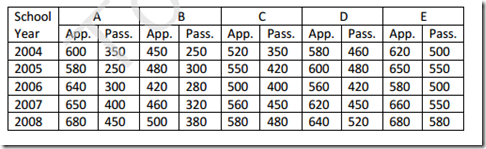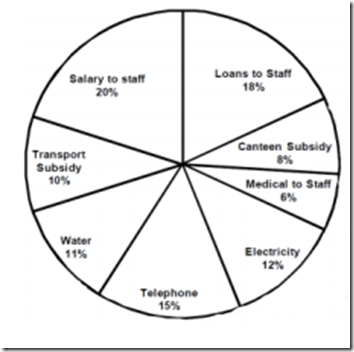Saturday, 13 May 2017

1 .

Q. (1 - 5):Study the following table carefully to answer these questions.
Number of students appeared and passed in an examination from five different schools over the years$Q.$ What is the ratio between the total numbers of students appeared from all schools together in the year 2004 and 2005 respectively?

 A.   286:295 B.   277:286 C.   286:277 D.   295:286
2 . During year 2006, which school had the highest percentage of students passed over appeared?

 A.   C B.   B C.   A D.   E
3 . For school D, which year had the lowest percentage of students passed over appeared?

 A.   2004 B.   2005 C.   2006 D.   2007
4 . What was the overall percentage of students passed over the number of students appeared from all the schools together in the year 2007? (round off to next inter)

 A.   74 B.   73 C.   76 D.   72
5 . What is the ratio between average number of students passed from schools B and C respectively for all the given years?

 A.   70:51 B.   70:53 C.   53:70 D.   51:70
6 .

Q. (6 - 10): Study the following graph carefully to answer these questions.
AVERAGE MONTHLY EXPENDITURE OF AN ORGANISATION UNDER VARIOUS HEADS TOTAL EXPENDITURE RS. 18,50,000.$Q.$ What is the difference between the expenditure on salary to staff and loans to staff?

 A.   Rs. 37,200/- B.   Rs. 35,700/- C.   Rs. 37,500/- D.   None of these
7 . What was the total expenditure on Electricity and water together?

 A.   Rs. 4,25,000/- B.   Rs. 4,25,500/- C.   Rs. 4,22,500/- D.   Rs. 4,25,800/-
8 . How much amount spent on Transport subsidy and canteen subsidy together?

 A.   Rs 3,34,000/- B.   Rs. 3,43,000/- C.   Rs. 3,30,000/- D.   Rs. 3,33,000/-
9 . Amount spent on medical to staff is what percentage of the amount spent on salary?

 A.   30% B.   33% C.   25% D.   22%
10 . How much amount spent on Telephone?

 A.   Rs. 2,75,500/- B.   Rs. 2,70,500/- C.   Rs. 2,77,500/- D.   Rs. 2,77,000/-
1 .
 Answer : Option B Explanation : Total no. of students appeared in 2004 from all the schools together.= 600 + 450 + 520 + 580 + 620= 2770Total no of students appeared in 2005 from all the school together= 580 + 480 + 550 + 600 + 650= 2860∴ Ratio = 2770 : 2860= 277:286
2 .
 Answer : Option D Explanation : Percentage of passed students in 2006 of school A.= $300\over 640$ x 100 = 46.88 %Percentage of passed students in 2006 of school B.= $280\over 420$ x 100 = 66.67 %Percentage of passed students in 2006 of school C.= $400\over 500$ x 100 = 80 %Percentage of passed students in 2006 of school D.= $420\over 560$ x 100 = 75 %% age of passed students in 2006 of school E. = $500\over 580$ x 100 = 86.21%∴ From the above calculation of all schools during the year 2006 school E have highest percentage of students passed over appeared.
3 .
 Answer : Option D Explanation : Percentage of passed students of School D in the year 2004 = $460\over 580$ \times 100Percentage of passed students of schools D in the year 2005 = $480\over 600$ x 100 = 80%Percentage of passed students of school D in the year 2006.= $420\over 560$ x 100 = 75%Percentage of passed students of school D in the year 2007= $450\over 620$ x 100 = 72.58%Percentage of passed students of school D in the year 2008.= $520\over 640$ x 100 = 81.25%∴ From the above calculation it is clear that in the year 2007, school D have lowest percentage of students passed over appeared.
4 .
 Answer : Option A Explanation : Total no. of students appeared in 2007.= 650 + 460 + 560 + 620 + 660= 2950Total no. of students passed in an examination in the year 2007.= 400 + 320 + 450 + 450 + 550 = 2170Therefore, $2170\over2950$ x 100 = 74%
5 .
 Answer : Option D Explanation : Average = $Total Number of students passed from school B \over Total Number of students passed from school C$= ${250+300+280+320+380\over5}\over{350+420+400+450+480\over5}$= ${1530\over5}\over{2100\over5}$= 306:420 = 102:140 = 51:70
6 .
 Answer : Option D Explanation : Expenditure on salary to staff expenditure on loans to staff.= Rs. 1850, 000 × $20\over 100$= Rs. 1850, 000 × $18\over 100$= Rs. 370,000 - 333000= Rs. 37000
7 .
 Answer : Option B Explanation : Expenditure on Electricity & water Together = Rs. 1850,000 × $11+12\over 100$= Rs. 1850,000 × $23\over 100$= Rs. 425500
8 .
 Answer : Option D Explanation : Amount spent on Transport subsidy & canteen subsidy together. = Rs. 1850000 x $10+8\over 100$= Rs. 1850,000 × $18\over 100$= Rs. 333000
9 .
 Answer : Option A Explanation : According to question,= ${$Rs.1850000 \times {6\over 100} \times 100$}\over {Rs. 1850000 \times {20\over 100}}$= $6\over 20$ x 100= 30%
10 .
 Answer : Option C Explanation : Amount spent telephone= Rs. 1850,00 × $15\over 100$= Rs. 277500/-